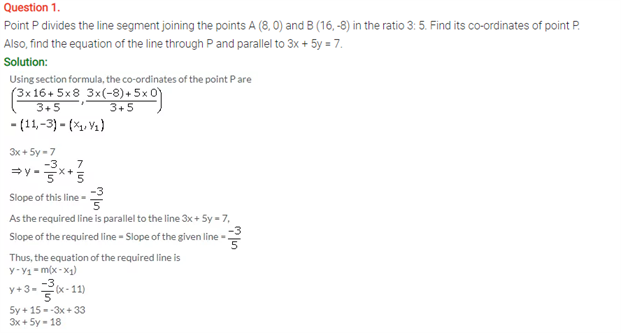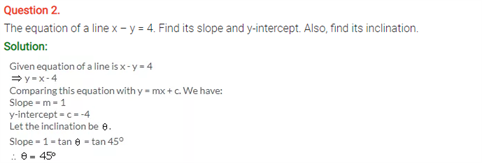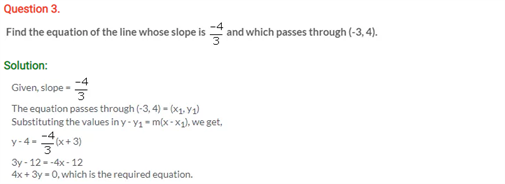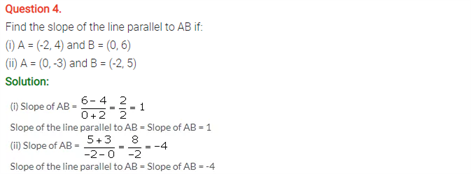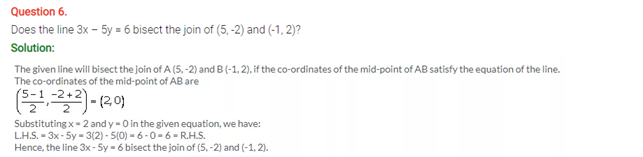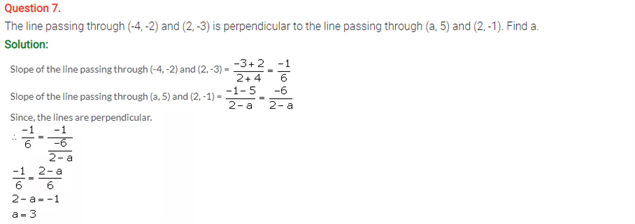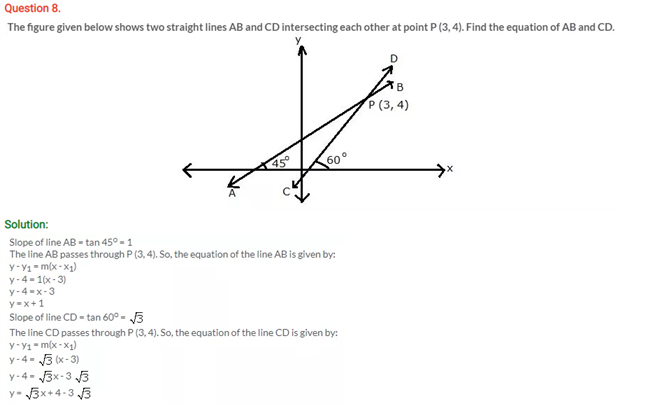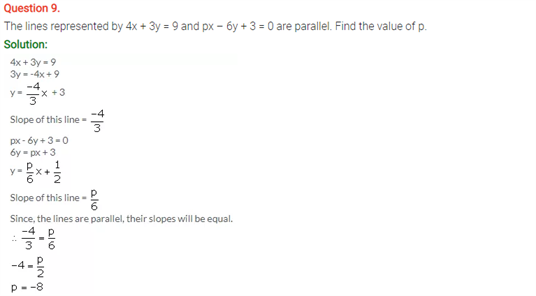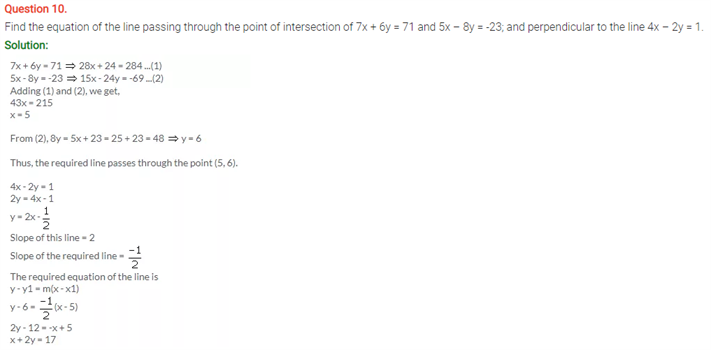# Selina Concise Maths Solution for ICSE Class 10 Chapter 14 Equation of a Line

## Selina Class 10 Maths Solutions for Chapter 14

The problems on the equation of a line can be found in ICSE Solutions For Class 10 Maths. In a line, certain formulas can describe the slope and coordinate plane. The formula y=mx+b defines the equation and x and y are the coordinates of the points whereas, m is the slope and b is the intersection of the line on the y-axis. These equations can be used to plot line diagrams with the mentioned measurements. Solving the solutions on this concept will ensure a better grasp of this chapter.

Students can find ICSE Class 10 Maths Selina Solutions Chapter 14 Equation Of a Line below: